# The logistic model¶

## Introduction¶

The logistic growth model is the differential equation: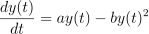for any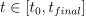, with the initial condition: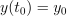where :

•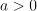and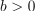are two real parameters,

•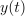is the size of the population at time,

•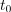is the initial time,

•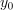is the initial population at time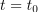,

•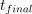is the final time.

Theparameter sets the growth rate of the population. Theparameter acts as a competition parameter which limits the size of the population by increasing the competition between its members.

In , the author uses this model to simulate the growth of the U.S. population. To do this, the author uses the U.S. census data from 1790 to 1910. For this time interval, R. Pearl and L. Reed  computed the following values of the parameters: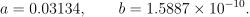Our goal is to use the logistic growth model in order to simulate the solution for a larger time interval, from 1790 to 2000: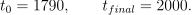Then we can compare the predictions of this model with the real evolution of the U.S. population.

We can prove that, if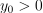, then the limit population is: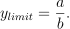In 1790, the U.S. population was 3.9 Millions inhabitants: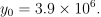We can prove that the exact solution of the ordinary differential equation is: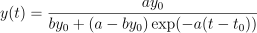for any.

We want to see the solution of the ordinary differential equation when uncertainties are taken into account in the parameters:

• the initial U.S. population,

• the parametersand.

Indeed, Pearl and Reed  estimated the parametersandusing the U.S. census data from 1790 to 1910 while we have the data up to 2000. Moreover, the method used by Pearl and Reed to estimate the parameters could be improved; they only used 3 dates to estimate the parameters instead of using least squares, for example. Finally, Pearl and Reed did not provide confidence intervals for the parametersand.

## Normalizing the data¶

The order of magnitude ofandare very different. In order to mitigate this, we consider the parameteras the logarithm of: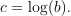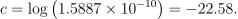The order of magnitude of the population is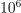. This is why we consider the normalized population in millions: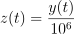for any.

Let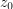be the initial population: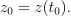## Uncertainties¶

Uncertainty can be accounted for by turning,andinto independent random variables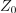,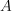and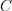with Gaussian distributions. From this point onward,,andrespectively denote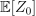,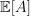and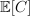.

Variable

Distributiongaussian, mean, coefficient of variation 10%gaussian, mean, coefficient of variation 30%gaussian, mean, coefficient of variation 30%

No particular probabilistic method was used to set these distributions. An improvement would be to use calibration methods to get a better quantification of these distributions. Calibration methods could be used to get a better quantification of these distributions.

## Variables¶

In the particular situation where we want to calibrate this model, the following list presents which variables are observed input variables, input calibrated variables and observed output variables.

• t: Input. Observed.

•, a, c: Inputs. Calibrated.

• z: Output. Observed.

We can load this model from the use cases module as follows :

>>> from openturns.usecases import logistic_model
>>> # Load the logistic model
>>> lm = logistic_model.LogisticModel()